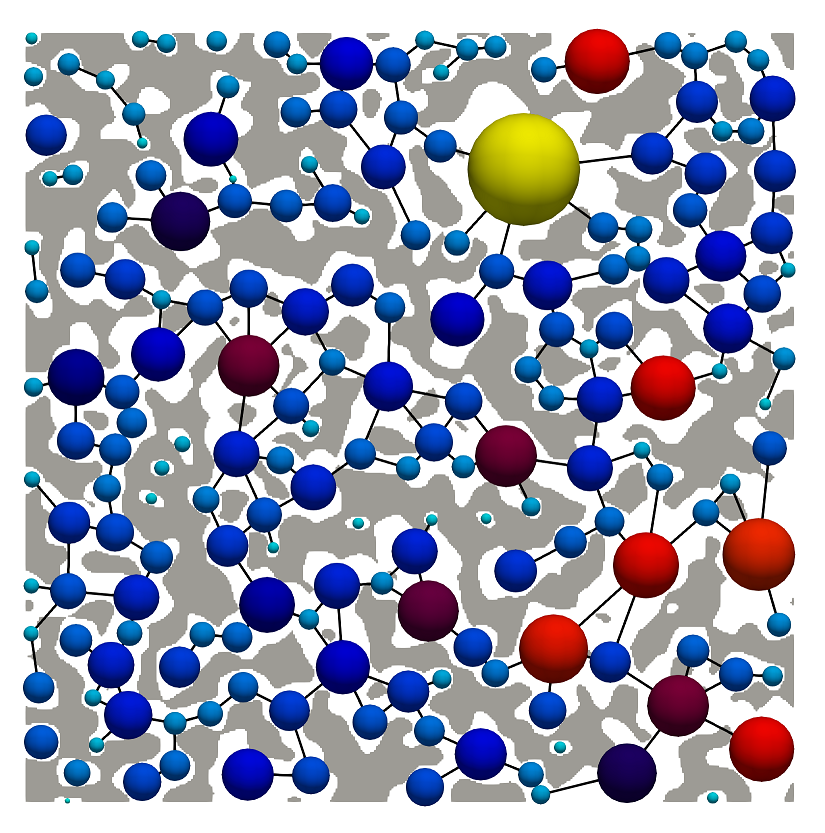# SNOW network extraction¶

The SNOW algorithm, published in Physical Review E, uses a marker-based watershed segmentation algorithm to partition an image into regions belonging to each pore. The main contribution of the SNOW algorithm is to find a suitable set of initial markers in the image so that the watershed is not over-segmented. SNOW is an acronym for Sub-Network of an Over-segmented Watershed. This code works on both 2D and 3D images. In this example a 2D image will be segmented using the predefined snow function in PoreSpy.

Start by importing the necessary packages:

:

import numpy as np
import porespy as ps
import openpnm as op
import matplotlib.pyplot as plt
ps.visualization.set_mpl_style()
np.random.seed(10)


Generate an artificial 2D image for illustration purposes:

:

im = ps.generators.blobs(shape=[400, 400], porosity=0.6, blobiness=2)
fig, ax = plt.subplots()
ax.imshow(im);SNOW is composed of a series of filters, but PoreSpy has a single function that applies all the necessary steps:

:

snow_output = ps.networks.snow2(im, voxel_size=1)


The snow function returns a python dict that is suitable for use in OpenPNM.

:

pn = op.network.GenericNetwork()
pn.update(snow_output.network)
prj = pn.project


OpenPNM has the ability to output network to a VTK file suitable for view in Paraivew:

:

prj.export_data(filename='extraction', filetype='vtk')


Finally, we can export the image in ‘vti’ format for visualization. PoreSpy offers a tool for this:

:

ps.io.to_vtk(np.array(im, dtype=int)[:, :, np.newaxis], 'im')


And the result after opening both files in ParaView is:You can also overlay the network on the image natively in porespy. Note that you need to transpose the image using im.T, since imshow uses matrix representation, e.g. a (10, 20)-shaped array is shown as 10 pixels in the y-axis, and 20 pixels in the x-axis.

:

fig, ax = plt.subplots()
ax.imshow(im.T);
op.topotools.plot_coordinates(fig=fig,
network=pn,
size_by=pn["pore.inscribed_diameter"],
color_by=pn["pore.inscribed_diameter"],
markersize=100)
op.topotools.plot_connections(network=pn, fig=fig)
ax.axis("off");